Next: Regularization in the wavelet Up: Deconvolution Previous: Deconvolution

Introduction

Consider an image characterized by its intensity distribution I(x,y), corresponding to the observation of an object O(x,y) through an optical system. If the imaging system is linear and shift-invariant, the relation between the object and the image in the same coordinate frame is a convolution:

 I(x,y)= O(x,y) * P(x,y) + N(x,y) (14.99)

P(x,y) is the point spread function (PSF) of the imaging system, and N(x,y) is an additive noise. In Fourier space we have: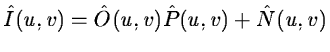(14.100)

We want to determine O(x,y) knowing I(x,y) and P(x,y). This inverse problem has led to a large amount of work, the main difficulties being the existence of: (i) a cut-off frequency of the PSF, and (ii) an intensity noise (see for example ).

Equation 14.99 is always an ill-posed problem. This means that there is not a unique least-squares solution of minimal norm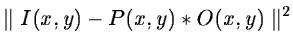and a regularization is necessary.

The best restoration algorithms are generally iterative . Van Cittert  proposed the following iteration: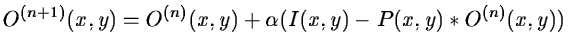(14.101)

where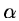is a converging parameter generally taken as 1. In this equation, the object distribution is modified by adding a term proportional to the residual. But this algorithm diverges when we have noise . Another iterative algorithm is provided by the minimization of the norm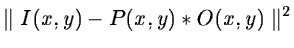and leads to: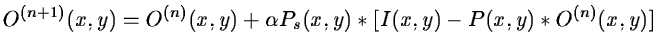(14.102)

where Ps(x,y)=P(-x,-y).

Tikhonov's regularization  consists of minimizing the term:(14.103)

where H corresponds to a high-pass filter. This criterion contains two terms; the first one,, expresses fidelity to the data I(x,y) and the second one,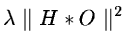, smoothness of the restored image.is the regularization parameter and represents the trade-off between fidelity to the data and the restored image smoothness. Finding the optimal value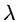necessitates use of numeric techniques such as Cross-Validation  .

This method works well, but it is relatively long and produces smoothed images. This second point can be a real problem when we seek compact structures as is the case in astronomical imaging. An iterative approach for computing maximum likelihood estimates may be used. The Lucy method [#lucy<#15258,#katsaggelos<#15259,#adorf<#15260] uses such an iterative approach:(14.104)

and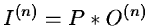(14.105)

where P* is the conjugate of the PSF.Next: Regularization in the wavelet Up: Deconvolution Previous: Deconvolution
Petra Nass
1999-06-15